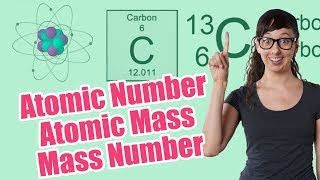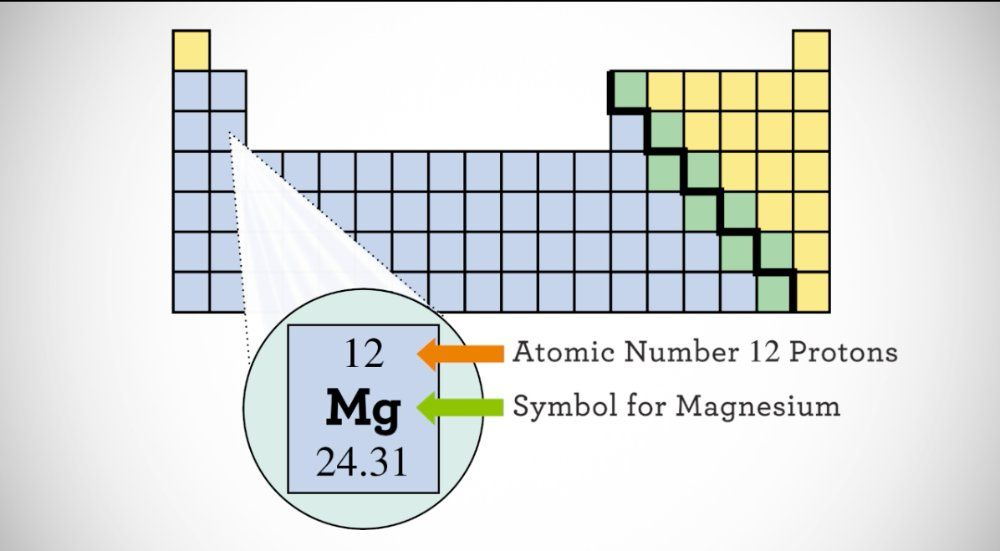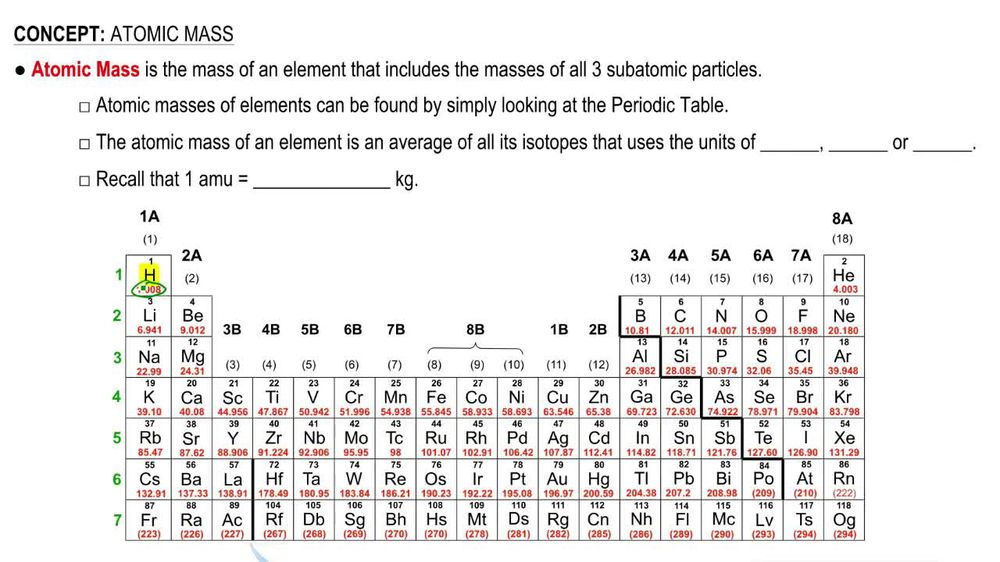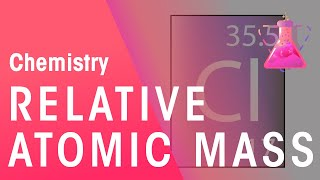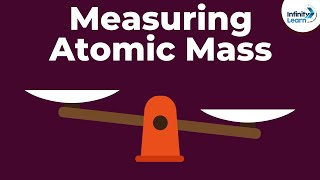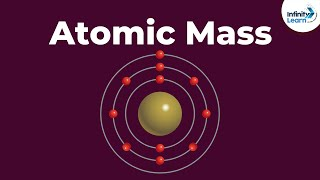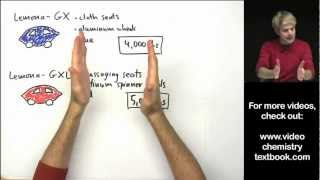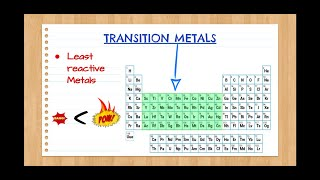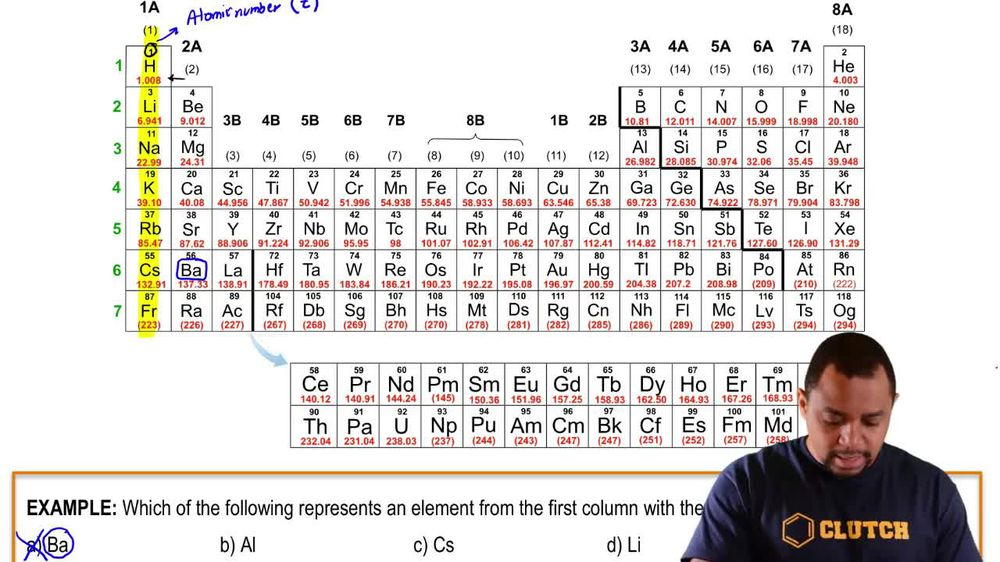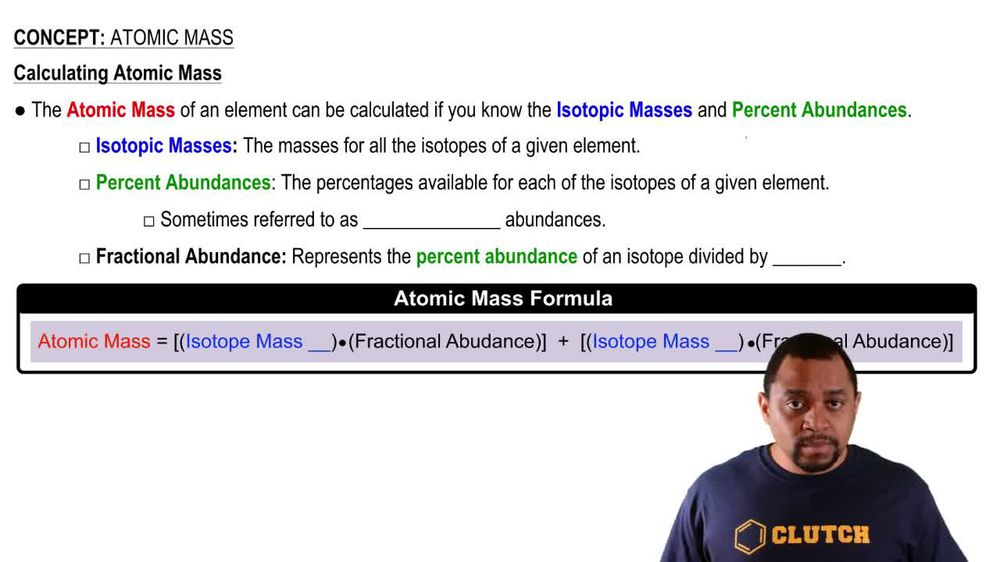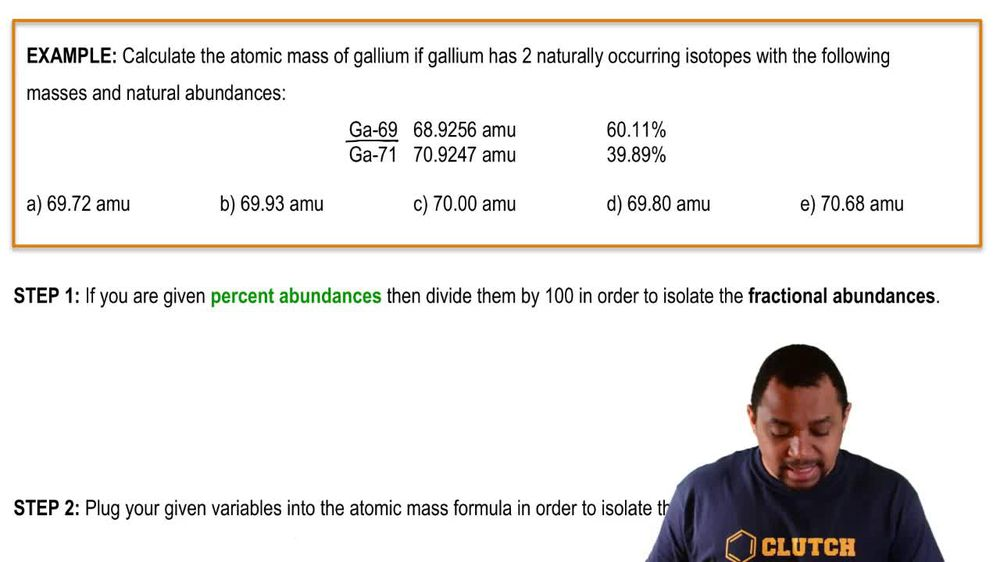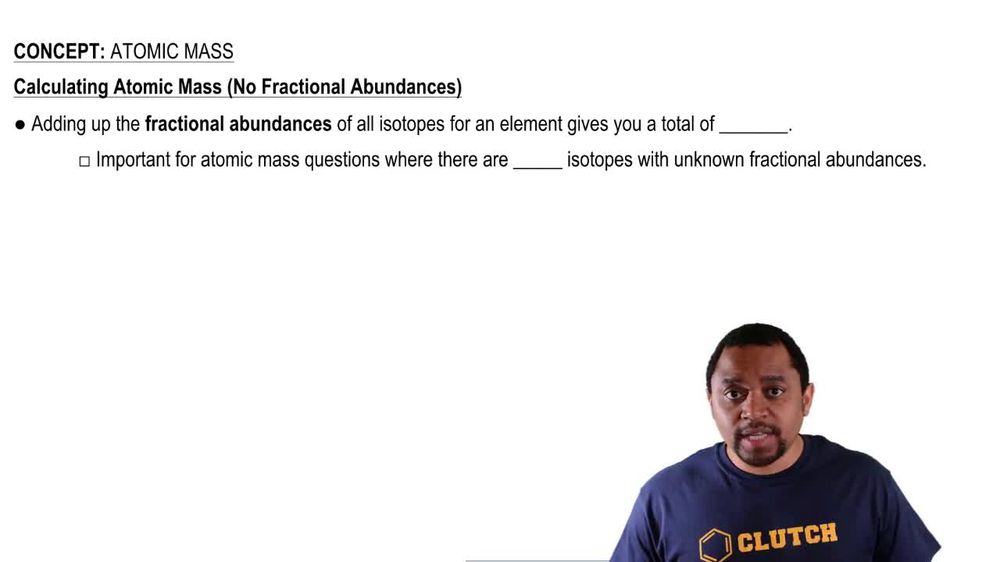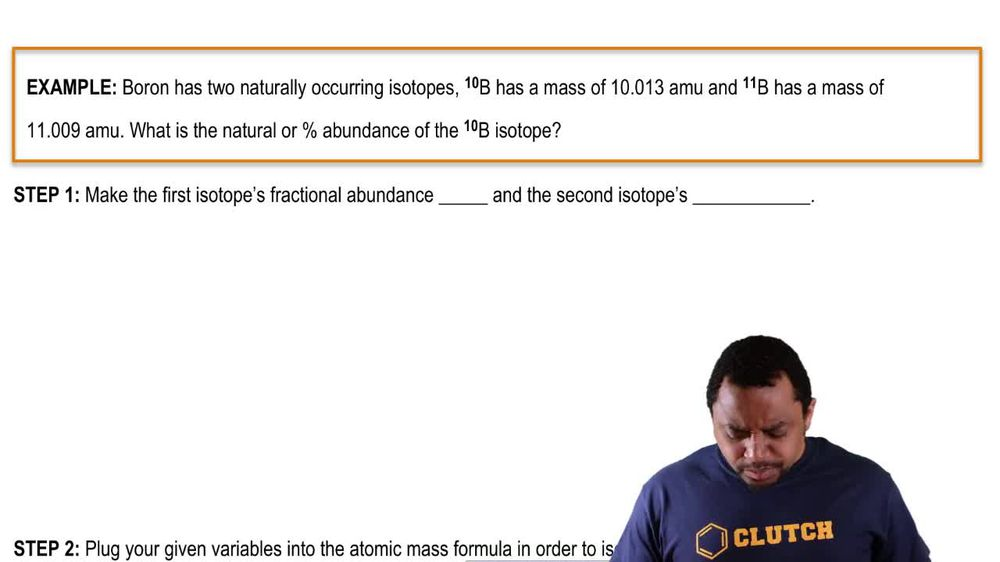Start typing, then use the up and down arrows to select an option from the list.
1. 2. Atoms & Elements2. Atomic Mass
Problem

# Naturally occurring iodine has an atomic mass of 126.9045 amu. A 12.3849 g sample of iodine is accidentally contaminated with an additional 1.00070 g of 129I, a synthetic radioisotope of iodine used in the treatment of certain diseases of the thyroid gland. The mass of 129I is 128.9050 amu. Find the apparent “atomic mass” of the contaminated iodine.

Relevant Solution3m
Play a video:
Hello everyone today. We are being given the following parliament asked to solve for it. It says Cesium has one stable isotope which has an atomic mass of 0.9054 A. M. U. A synthetic radioisotopes. Cesium 1 37. With a mass of 1.2410 g was accidentally added to 13.2541 g of cesium sample. The atomic mass of 1 37 cesium is 136.9071 A. M. You what is the apparent molecular mass of the contaminated cesium? So first we have to take into account our total mass which is going to be the 1.02410 g From our Cesium 1 37. That's also going to be our 13. grams of our original sample. And this is going to give us a total of 14.278g of a sample of our total mess. And so to find the fraction of our original cesium We have to sort of find the fraction of our original cesium. We have to take our 13.2541 or our mass of that cesium and divided by the total amount that we have. This is going to give us 0.9-8. And to find the fraction Of our 1 37 cesium. We're gonna take that mass or 1.02410 g. And divide that by the total mass which is 14.278g. That is going to give us 0.07172 as our final answer for that. 3rdly, we want to calculate the atomic mass am I doing that? We're gonna go ahead and take our mass, our original mass of our original cesium. And we're gonna multiply that by the fraction. So you multiply that by the fraction of our isotope one. And we're going to add that to the mass of our contaminant or cesium 1 37. And multiply that by the fraction which is isotope too. And so that's going to look like our 1 32.9 A. M. U. Times our 0.9 to eight plus our 1 36.9071 A. M. You Multiplied by our 0.0717. And that is going to give us an atomic mass of 133.1 92 A. M. U. As our final answer, I hope this helps. And until next time